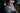###Eric Jinks — June 18th, 2018

A useful feature of TensorFlow.js is the ability to convert and load pre-existing models developed in python with Keras or TensorFlow for browser use. This gives us potential improvements in performance for end-users by making use of their device GPU to conduct the inference without network bottlenecks.

To demonstrate, I'm using a convolutional neural network classifier created in python using Keras and TensorFlow. The classifier was trained on 8000 labelled images of cats and dogs which were pre-processed with data augmentation.

The model was then exported to HDF5 (.h5) format and converted to TensorFlow.js Layers format using the `tensorflowjs_converter` cli tool. I've included the model source code at the end of this post.

``const model = await tf.loadModel('/path/to/model.json')``

To run a prediction on new image data, we make use of the `tf.fromPixels()` method. Using this we can draw image data from sources such as file uploads, streaming video from webcams / device cameras and HTML canvas elements. In this demo, you can add use and example image or upload you own.

``````const predict = async imageSource => {
const img = document.createElement('img')
img.src = imageSource
img.onload = async () => {
const example = tf.fromPixels(img)
const newImage = tf.cast(
tf.image.resizeBilinear(example, [128, 128]),
'float32'
)
const norm = tf.fill([128, 128, 3], 255)
const normalisedImage = tf.div(newImage, norm)
const predictme = tf.cast(tf.expandDims(normalisedImage), 'float32')
const prediction = model.predict(predictme)
const timeStart = new Date()
const classificationData = await prediction.data()
const timeEnd = new Date()
const duration = timeEnd - timeStart
const classificationName = classificationData > 0.5 ? 'Dog' : 'Cat'
const confidence =
Math.round(Math.abs(classificationData - 0.5) * 2 * 100) + '%'
return { classificationName, classificationData, confidence, duration }
}
}``````

### The pre-trained model source

``````# Convolutional Neural Network
from keras.models import Sequential
from keras.layers import Convolution2D, MaxPooling2D, Flatten, Dense
classifier = Sequential()

# Convolution
convolution_layer = Convolution2D(filters=32, kernel_size=(3, 3), input_shape=(128, 128, 3), activation='relu')

# Max Pooling
pooling_layer = MaxPooling2D(pool_size=(2, 2))

# Add a second convolution layer
convolution_layer_2 = Convolution2D(filters=32, kernel_size=(3, 3), activation='relu')
pooling_layer_2 = MaxPooling2D(pool_size=(2, 2))

# Flattening
flattening_layer = Flatten()

# Full Connection
hidden_layer = Dense(units=256, activation='relu')
output_layer = Dense(units=1, activation='sigmoid')

# Compile

# Data pre-processing
from keras.preprocessing.image import ImageDataGenerator

train_datagen = ImageDataGenerator(
rescale=1./255,
shear_range=0.2,
zoom_range=0.2,
horizontal_flip=True)

test_datagen = ImageDataGenerator(rescale=1./255)

training_set = train_datagen.flow_from_directory(
'dataset/training_set',
target_size=(128, 128),
batch_size=32,
class_mode='binary')

test_set = test_datagen.flow_from_directory(
'dataset/test_set',
target_size=(128, 128),
batch_size=32,
class_mode='binary')

classifier.fit_generator(
training_set,
epochs=25,
validation_data=test_set)

# Export model
from datetime import datetime
i = datetime.now()
filename = f'cnn_model_{i.strftime("%Y-%m-%d-%H-%M-%S")}.h5'
classifier.save(filename)
print(f'Saved file: {filename}')``````

See the TensorFlow.js docs for more info. Example photos by Mia Phoy and Freddie Marriage.